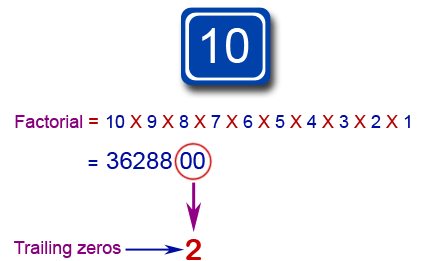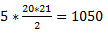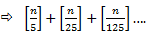# How to Find Number of Trailing Zeros in a Factorial or Product Notes | Study Quantitative Aptitude for GMAT - GMAT

## GMAT: How to Find Number of Trailing Zeros in a Factorial or Product Notes | Study Quantitative Aptitude for GMAT - GMAT

The document How to Find Number of Trailing Zeros in a Factorial or Product Notes | Study Quantitative Aptitude for GMAT - GMAT is a part of the GMAT Course Quantitative Aptitude for GMAT.
All you need of GMAT at this link: GMAT

Trailing zero is a zero digit in the representation of a number which has no non-zero digits that are less significant than the zero digit. Put more simply, it is a zero digit with no non-zero digits to the right of it.Representation of Trailing Zeros(i) Number of trailing zeroes in a Product or Expression
If we look at a number N, such that
N = 170130000 = 17013*104

Number of trailing zeroes is the Power of 10 in the expression or in other words, the number of times N is divisible by 10.
For a number to be divisible by 10, it should be divisible by 2 & 5.
Consider a number N, such that
N = 2a * 5b * x
For the number to have a zero at the end, both a & b should be at least 1.
If you want to figure out the exact number of zeroes, you would have to check how many times the number N is divisible by 10.
When I am dividing N by 10, it will be limited by the powers of 2 or 5, whichever is lesser.
Number of trailing zeroes is going to be the power of 2 or 5, whichever is lesser.
Let us look at an example to further illustrate this idea.

Q.1. What is number of trailing zeroes in 12000?
Ans. 12000 = 25 * 3 * 53
When I divide it by 10, it would be divisible exactly thrice because I have only three 5s.
In this case, number of 5s has become the limiting factor and so, the power of 5, which is 3 is the answer.
Tip: The power of 5 will be the limiting factor in most cases of continuous distribution. It will happen because 5 is less likely to occur than 2.

Q.2. Find out the number of zeroes at the end of N?
Ans.  N = 11 * 22 * 33 .... .100100
Looking at the expression, we can say that the power of 5 will be the limiting factor.
All we need to do is to figure out the number of 5s in the expression.
11, 22, 33, 1717, 8989,… will not give us any 5s.
55 will give us five 5s.
1010 will give us ten 5s.
1515 will give us fifteen 5s.
And so on.
So, the total number of 5s that I have is
⇨ 5 + 10 + 15 ....100 = 5(1+2+3...20) =But I have made a mistake in the above calculation.
I have assumed that 2525 will give me twenty-five 5s but that is incorrect.
It is incorrect because 2525 = 550 and will actually give me 50 5s.
Other errors are:
5050 will actually give me 100 5s, whereas I have considered only 50 5s.
7575 will actually give me 150 5s, whereas I have considered only 75 5s.
100100 will actually give me 200 5s, whereas I have considered only 100 5s.
Considering the above, I have made an error of = 25 + 50 + 75 + 100 = 250 5s.
So the total number of 5s that I have are 1050 + 250 = 1300.
So the number of trailing zeroes at the end of the expression is 1300

(ii) Number of trailing zeroes in a factorial (n!)
Number of trailing zeroes in n! = Number of times n! is divisible by 10 = Highest power of 10 which divides n! = Highest power of 5 in n!
The question can be put in any of the above ways but it can be answered using the simple formula given below:{[x] is the greatest integer function. [4.99] = 4, [4.01] = 4, [- 4.99] = 5, [-4.01] = 5}
The above formula gives us the exact number of 5s in n! because it will take care of all multiples of 5 which are less than n. Not only that it will take care of all multiples of 25, 125, etc. (higher powers of 5).

Tip: Instead of dividing by 25, 125, etc. (higher powers of 5); it would be much faster if you divided by 5 recursively.

Q.3. What is the number of trailing zeroes in 23!
Ans. [23/5] = 4. It is less than 5, so we stop here.

Q.4. What is the number of trailing zeroes in 123!
Ans. [123/5] = 24
Now we can either divided 123 by 25 or the result in the above step i.e. 24 by 5.
[24/5] = 4. It is less than 5, so we stop here.
The answer is = 24 + 4 = 28

Q.5. What is the number of trailing zeroes in 1123!?
Ans. [1123/5] = 224
[224/5] = 44
[44/5] = 8
[8/5]=1. It is less than 5, so we stop here.
The answer is = 224 + 44 + 8 + 1 =277

FINDING OUT 'N' WHEN NUMBER OF TRAILING ZEROS IS KNOWN
Q.6. Number of trailing zeroes in n! is 13. n = ?
Ans. There is no standard formula for such type of questions but they can be solved by a little bit of hit and trial.
I need to get 13 trailing zeroes which I will definitely get from 65!
But it will have some extra zeroes in the end because of higher powers of 5.
So, I will consider the previous multiple of 5, which in this case is 60.
Trailing zeroes in 60! = [60/5] + [60/25] = 12 + 2 = 14
I got 14 but I want to get 13, so I will consider the previous multiple of 5, which in this case is 55.
Trailing zeroes in 55! = [55/5] + [55/25] = 11 + 2 = 13
So, the valid values of n! are 55!, 56!, 57!, 58!, 59!

Q.7. Number of zeroes in n! is 23. n =?
Ans. Trailing zeroes in 100! = [100/5] + [100/25 ] = 20 + 4 = 24 {Too high. Consider previous multiple}
Trailing zeroes in 95! = [95/5] + [95/25] = 19 + 3 = 22 {Too low. Consider next multiple}
As you can see from above, we would end up in a loop.
This will happen because there is no valid value of n for which n! will have 23 zeroes in the end.

The document How to Find Number of Trailing Zeros in a Factorial or Product Notes | Study Quantitative Aptitude for GMAT - GMAT is a part of the GMAT Course Quantitative Aptitude for GMAT.
All you need of GMAT at this link: GMATUse Code STAYHOME200 and get INR 200 additional OFF

## Quantitative Aptitude for GMAT

75 videos|87 docs|156 tests

Track your progress, build streaks, highlight & save important lessons and more!

,

,

,

,

,

,

,

,

,

,

,

,

,

,

,

,

,

,

,

,

,

;